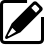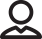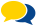## Spring Design

Design information for engineers, such as spring calculation formulas,

which are the basis of spring design, can be found here.

### CompressionSprings

For example, when the following conditions are given, the material diameter, number of active coils, spring constant, and stress can be calculated as follows.
When P = 9,000 N, we will design a compression coil spring of = 30 mm, = 290 Mpa, D = 100 mm, Hf = 200 mm. The permissible stress is 550 Mpa or less.Therefore, the stress of this spring at 9,000 N is equal to or less than the permissible stress.Product informationOur ProposalsPopular questions